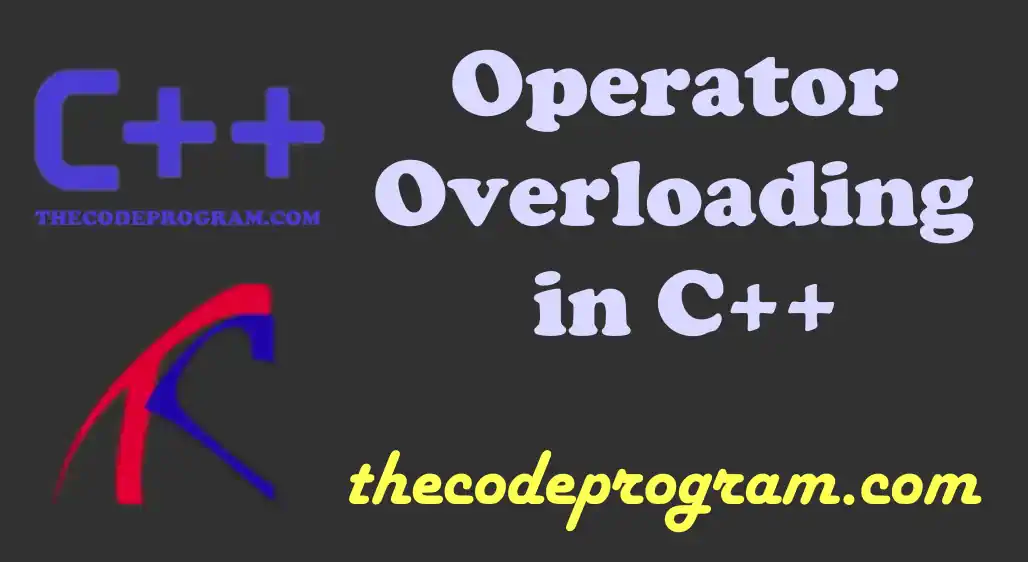Let's begin

Operator overloading is adding more user-defined functionalities to operators and letting them to work as user defined functions.

We can overload the assigned function and redefine the functionality of related operator. For example we can combine two strings with plus operator. We can redefine this function and redefine according to our requirement.

``````
returningType operator symbolOfOperator(parameter) {
}
``````
• returningType Returning data type of overloaded operator function.
• operator operator is keyword. We need to use as directly as it is.
• symbolOfOperator What operator symbol will be overloaded. (++, --, *, etc...)
• parameter What data will it take and process.

Now let's make an operator overloading example for better understanding. In this example I created two classes Square and Circle. I will make somre operations with overloaded operators.

Below code block you can see the Square class:
``````
class Square {
private:
int area;
public:
Square(double _area = 0)  {area = _area; }
void printArea() { cout << area << endl; }
Square operator+(const Square& _s) {
Square s;
return s.area = this->area + _s.area;
}
};
``````

We will initialzie this class with a specified area value. In this class we overloaded (+) operator for summing areas of two different squares.

Below code block you will see How to use the overloaded operator
``````
Square sq1(50), sq2(90);
cout << "Area of Square 1 is  ";
sq1.printArea();
cout << "Area of Square 2 is  ";
sq2.printArea();
``````
Program output will be:
``````
Area of Square 1 is  50
Area of Square 2 is  90
Summarized area is 140
``````

Another example class have more overloadings. In here we overloaded three operators. We have much more operations in this class.

Below you can see the Circle class.
``````
class Circle {
private:
const double PI = 3.14;
double area;
void calculateArea(){
}
public:
this->calculateArea();
}
void printData() {
cout << "Radius is " << radius << " and Area is :" << area  << endl;
}
void operator |=(const double _rad) {
this->calculateArea();
}
void operator +=(const double val) {
this->calculateArea();
}
void operator %=(const double _area) {
this->area = _area;
this->calculateArea();
}
};
``````
In this class we overloaded operators:
• |= To set the radius directly.
• += To increase the radius with specified value.
• %= To set the area of circle directly and calculate the new radius according to new area.
In all overloaded operators we re calculated the area of circle.
Below code block you will see how did we use this operators:
``````
Circle circle(10);
circle.printData();
circle |= 25;
circle.printData();
circle += 33.965;
circle.printData();
circle %= 314000;
circle.printData();
``````
Example Output will be
``````
Radius is 10 and Area is :314
Radius is 25 and Area is :1962.5
Radius is 58.965 and Area is :10917.4
Radius is 316.228 and Area is :314000
``````

As you can see above example we overloaded |= , += and %= operators. We set some values and calculated the related datas according to these values.

There are some rules that we can not overload some operators. These operators are belong to programming language and can not be overloaded.
• :: Class scope specifier operator
• . Class member calling operator
• .* Pointer specifier operator
• ?: Operator of ternary operattion

Burak Hamdi TUFAN

20/10/2021

10/07/2022

08/09/2023